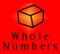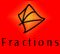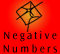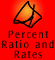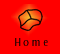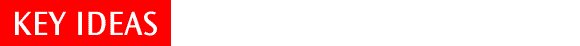| Place Value | Comparing Decimal Numbers |
| Importance of the zero in place value | Rounding numbers | Quick Quiz |

Place Value

Like whole numbers the place of the digit within a decimal number represents the value of that digit.

The name of each column and the value it represents is shown below. This chart continues endlessly in both directions. We call it the "endless base ten chain." As has been established with whole numbers there are numbers much larger than thousands and consequently there are numbers much smaller than thousandths.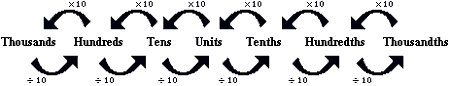Each place value or column to the left is ten times larger than the one to the right. For example, 1 hundred is ten times 1 ten (10 x 10=100); and 1 tenth is 10 times 1 hundredth (10 x 0.01= 0.1). This is especially important to understand when multiplying and dividing with decimal numbers and will be discussed further in the relevant sections.

Within a number, such as 3.097 each of the digits has a different value depending upon its place.

 Ones Tenths Hundredths Thousandths 3 0 9 7

The number can be written in its extended form as 3 ones, 9 hundredths and 7 thousandths. Note it is not necessary in this case to say that there are zero tenths, but the zero tenths is of great significance in the symbolic recording. If the zero was omitted and the number was written as just 3.97, its value would be changed. The 7 would be 7 hundredths instead of 7 thousandths.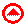Comparing decimal numbers

Comparing the size of decimals is easier than comparing fractions. This is an important advantage.

Method 1. Compare by relating to common fractions.

To compare 2.345 and 2.9, think of them as 2 and 345 thousandths and 2 and 9 tenths. Convert both to thousandths - which is easy because of the base 10 system!

2.345 = 2 and 345 thousandths = 2.345

2.9 = 2 and 900 thousandths = 2.900

900 is greater than 345 so 2.345 is greater than 2.9

As this example shows, this method amounts to adding zeros to the right hand side of the shorter number (which does not change its value) until the lengths are equal and then comparing as whole numbers

Method 2. Comparing digits from left to right.

This method parallels the usual method for comparing the size of whole numbers. As with whole numbers, when comparing decimal numbers it is important to always start by comparing from the highest place value column and moving to the smallest.

For example, in comparing 141 and 120 both numbers have 1 hundred. The first number has 4 tens and the second has 2 tens. 4 tens is larger than 2 tens so it is the larger number. No number in the 120's is as big as 130, and so certainly not as big as 140.

In 2.34569 and 2.9 the highest place value is ones. Both numbers have two ones. Next compare the tenths. There are 3 tenths in the first number and 9 tenths in the second number. 9 tenths is larger than 3 tenths so 2.9 is the larger of the two numbers, even though it has fewer digits than 2.34569.

Why does this method work? All the numbers that begin with 2.3XXXXX are less than 2.4. The biggest with various number of decimal places are 2.399, 2.3999, 2.39999999999 etc. All of these are less than 2.4, so must be less than 2.9.

(Additional note: There is one exception to the above. The number 2.39 repeated is actually equal to 2.4)Importance of the zero in place value

The place of the zero in all numbers is important. Adding zeros to the end of a number with a decimal point helps in organising the place value of the digits and it does not change the value of the number.

 For example 2.37 = 2 + 3 tenths + 7 hundredths and 2.370 = 2 + 3 tenths + 7 hundredths + 0 thousandths so 2.37 = is equal to 2.370

This is useful in algorithms and when comparing numbers.

Any number of zeros can be added: 2.37 = 2.370 = 2.37000 = 2.3700000 etc

Example: which is larger, 2.37 or 2.376?

Write 2.37 as 2.370,

 Ones Tenths Hundredths Thousandths 2 3 7 6 2 3 7 0

The top number (2.376) has six thousandths and the bottom number (2.37 = 2.370) has zero thousandths. Therefore the top number is six thousandths larger than the bottom number.Rounding Numbers

Rounding numbers involves finding the closest number ending in the required place value unit. As with whole number rounding, the degree of rounding will depend upon the degree of accuracy required. This may involve rounding to the nearest one, tenth, hundredth and so on, depending on what is needed.

There is a simple rule, (e.g. identify the digit after the relevant place value column; if it is 5 or above, then round up, otherwise round down) but it is important to understand the process involved.

a) rounding 1.8 to the nearest one

In this example, the closest numbers of the required place value unit are 1 and 2. The diagram shows 1.8 in comparison to 1 and 2.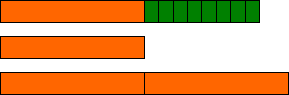We can see that 1.8 is closer to 2 than it is to 1. So 1.8 rounded to the nearest one is 2.

b) rounding 0.33 to the nearest tenth

In this example, the nearest numbers of the tenths place value unit are 0.3 and 0.4.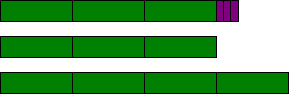We can see that 0.33 is closer to 0.3 than it is to 0.4. So 0.33 rounded to the nearest tenth is 0.3.

c) rounding if the number is exactly halfway

The number 2.65 is exactly half way between 2.6 and 2.7, so how can we round it to the nearest tenth? Does it go up or down? There is no reason to choose one over the other, so people have simply decided to go up. To round 2.65 to the nearest tenth, the answer is therefore 2.7.Key Ideas Quiz

1. Round 297.518 to the nearest:

 (a) tenth (b) ten (c) hundredth

2. Round 0.86725 to the nearest:

 (a) thousandth (b) one (c) hundredth (d) ten thousandth